# Coxeter groups and the ADE classification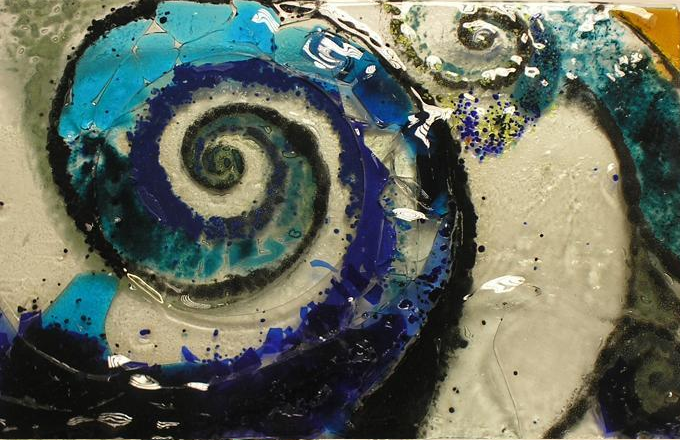and consider a reflection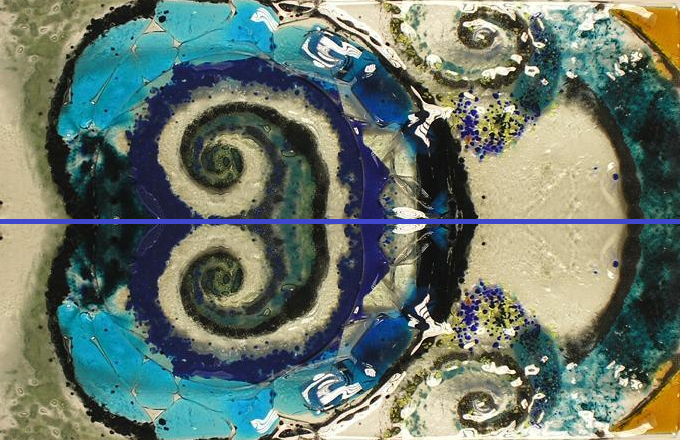Each reflection is represented by a point in the Coxeter-Dynkin diagram. So a single reflection will be represent by just a single point.Next consider two reflections with their mirrors at right-angles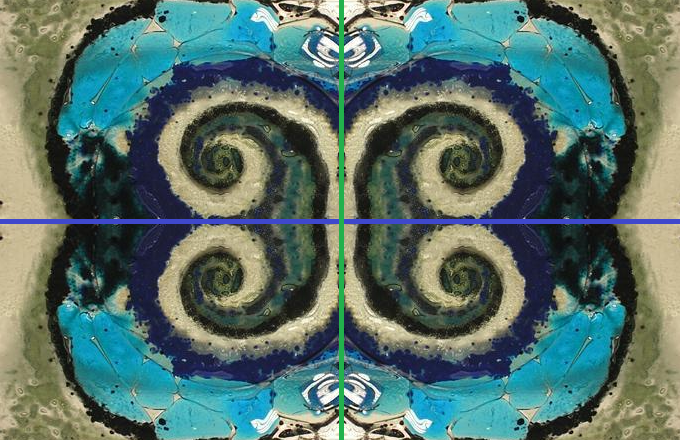if you do one followed by the other you get a rotation by 180°. If you do this twice you get back to the beginning, so the combined operation has order 2. This is the typical arrangement so for the CD diagram we just draw this as two points without a line between them.What if the two reflections are at 60° then performing the reflections one after the other gives a rotation by 120° this has order 3. You also get a third reflection (in yellow) by doing the first reflection, then the second and then the first reflection again.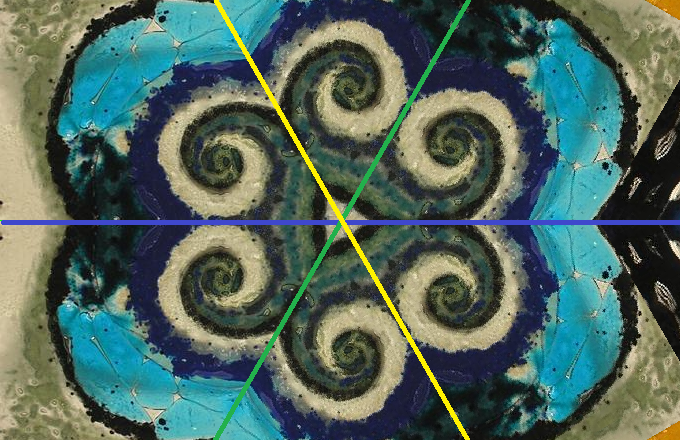An important point is the difference between elements and generators of the group. For the 60° reflection there are six elements in the group: the identity, reflection about 0° (in blue), reflection about +60° (in green), reflection about -60° (in yellow), rotation +120° rotation of -120°. Two of the reflections are chosen as generators as the third can be found by combining the other two. In the CD has two points with a single line joining them.If they are at 45° then the two reflections can be combined to give two other reflections (in yellow and orange) and rotations by 90°, 180° and 270°.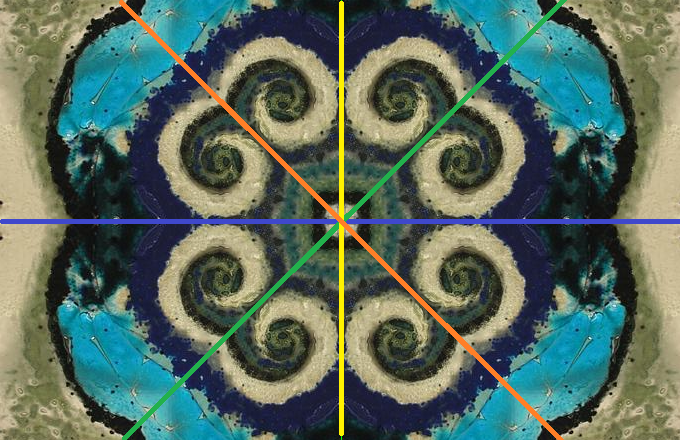the rotation has order 4 and the CD diagram has two point joined by a double lineor a line with index 4.We could consider other angle. Two reflections at 36° give a five-fold rotation this would be shown as a triple line or a line with index 5. Two reflections at 30° give a six-fold rotation with a quadruple line joining the points in the CD diagram. For what follows we are less interested in these higher fold reflections.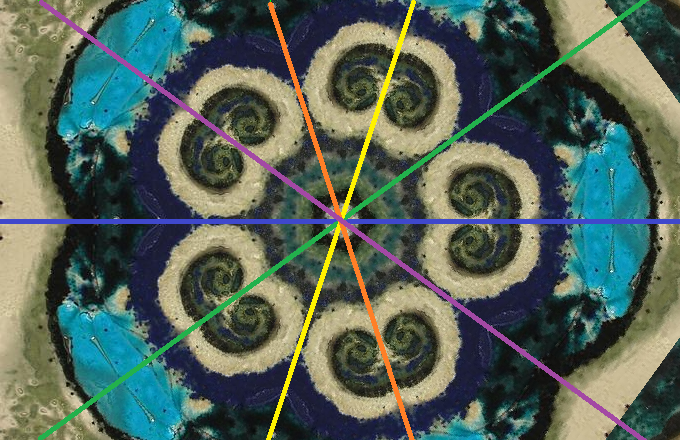Description Diagram Notes Single generatorTwo generator of order 2For example two reflections at 90°. Doing the first reflection followed second gives the same as the second followed by the first, so these are said to commute, are draw with no single line joining them. Two generator of order 3e.g. two reflections 60°. Joined with a single line. Two generator of order 4e.g. two reflections at 45°. Joined with line with index 4. Two generator of order 5e.g. two reflections at 36°. Joined with line with index 5. Two generator of order 6e.g. two reflections at 30°. Joined with line with index 6.

For other groups we need to consider the symmetry of objects in higher dimensions. To get to A3 we can consider the symmetries of the tetrahedron. There are six possible reflection, one for each edge, each reflection swaps two of the vertices. We just need three of these to generate the whole group. Here is a tetrahedron with those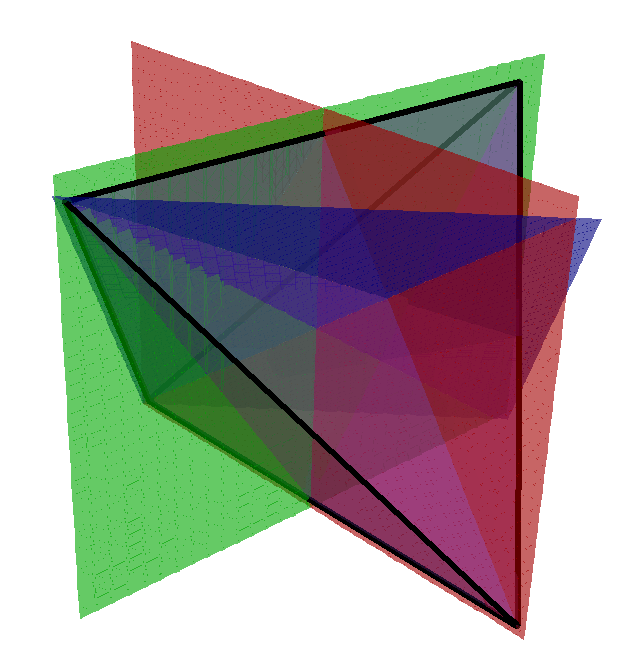3D Model

and just the reflections.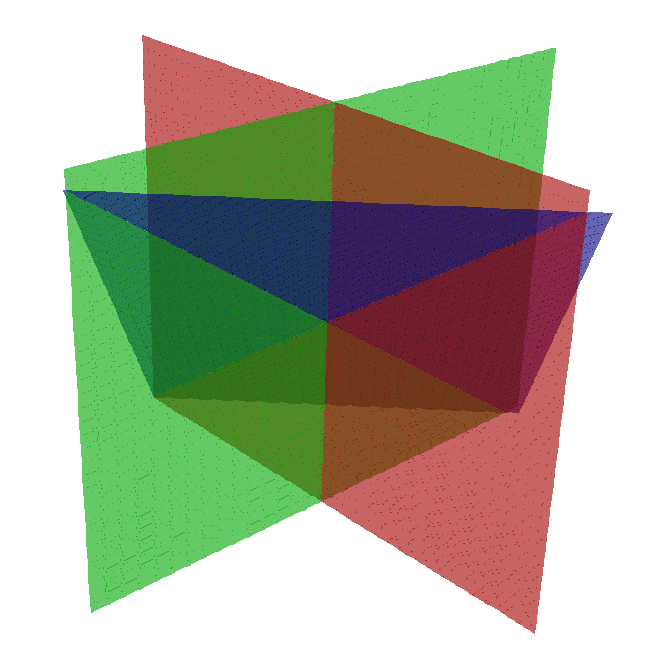The arrangement of these reflections is slightly odd. Two (red and green) are at right angles to each other the other pairs (red-blue, green-blue) are at 60°. In the CD diagram there are three nodes red, blue, green. As the red green reflection have order 2 they have no lines between them, the red-blue and blue-green pairs are both of order 3 so have a single line joining themWe could generalize this in two ways: going up a dimension. The 4D version of a simplex is the 5-cell with 5 points, 10 edges, 10 faces, 5 tetrahedra. This give the group A4 generated by four reflections, A, B, C, D. A-B are at 60° as are B-C and C-D. The other pairs of reflections A-C, A-D, B-D are at right angle so no line in the CD and the full CD is:We could also consider other 3D polyhedra, the cube can be generated by three reflection.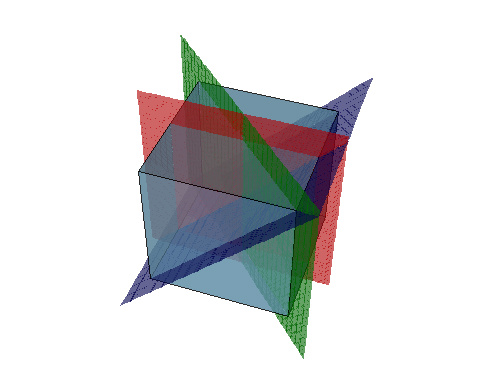3D Model

Red and Green are at 45° so have a double line in the CD Green and Blue are at 60° so have a single line Blue and Red are at right angles so have no line in the CD. The full CD isTo get to the interesting systems like D4, we need to consider 4D polytopes. The demi-tesseract has a D4 structure. This will have four reflection A B C D one of these B will at 60° to the other three reflections. The other combinations will be at right-angles.

To understand this group lets return to the symmetry of the tetrahedron. Take a tetrahedron centred at the origin with vertices $a=(1,-1,1), b=(-1,1,1), c=(1,1,-1), d=(-1,-1,-1)$. The three reflections used as generators are $$G=\begin{pmatrix}0 & 1 & 0\\ 1 & 0 & 0 \\ 0 & 0 & 1\end{pmatrix}, R=\begin{pmatrix}0 & -1 & 0\\ -1 & 0 & 0 \\ 0 & 0 & 1\end{pmatrix}, B=\begin{pmatrix}1 & 0 & 0\\ 0 & 0 & 1 \\ 0 & 1 & 0\end{pmatrix}$$ $G$ swaps the first two coordinates and sends $a\to b$, $b\to a$, $c\to c$, $d\to d$, $R$ swaps and negates the first two coordinates sends $a\to a$, $b\to b$, $c\to d$, $d\to c$, $B$ swaps the last two coordinates sends $a\to c$, $b\to b$, $c\to a$, $d\to d$. See full list of elements.

To go to four dimensions we have a Demitesseract with vertices of two copies of a tetrahedron $a=(1,-1,1,1), b=(-1,1,1,1), c=(1,1,-1,1), d=(-1,-1,-1,1)$, $a'=(-1,1,-1,-1), b'=(1,-1,-1,-1), c'=(-1,-1,1,-1), d'=(1,1,1,-1)$.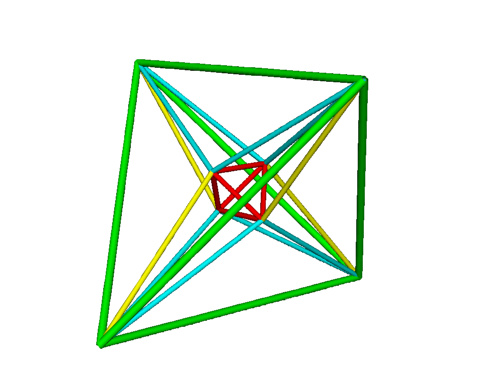A projection of the 4D 16-cell to 3D. The red tetrahedron has vertices $a, b, c, d$ with $w=1$, the green tetrahedron has vertices $e, f, g, h$, with $w=-1$. The yellow and cyan lines join the inner tetrahedron to the outer one.

The generators are the same as for A3 which also leaves the last coordinate unchanged

$$G=\begin{pmatrix}0 & 1 & 0 & 0\\ 1 & 0 & 0 & 0\\ 0 & 0 & 1 & 0 \\ 0 & 0 & 0 & 1\end{pmatrix}, R=\begin{pmatrix}0 & -1 & 0 & 0\\ -1 & 0 & 0 & 0\\ 0 & 0 & 1 & 0 \\ 0 & 0 & 0 & 1\end{pmatrix}, B=\begin{pmatrix}1 & 0 & 0 & 0\\ 0 & 0 & 1 & 0\\ 0 & 1 & 0 & 0 \\ 0 & 0 & 0 & 1\end{pmatrix}$$ plus a forth that swaps the last two coordinates $$Y=\begin{pmatrix}1 & 0 & 0 & 0\\ 0 & 1 & 0 & 0\\ 0 & 0 & 0 & 1 \\ 0 & 0 & 1 & 0\end{pmatrix},$$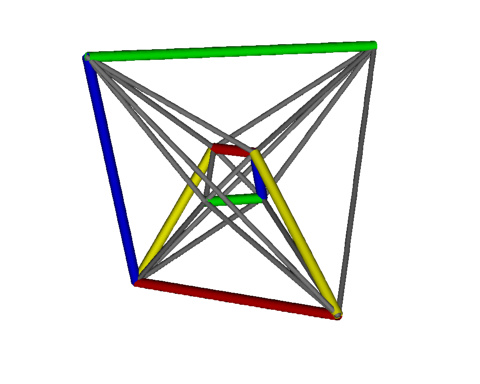Edges coloured to show action of the group generators.

Looking at the action of the generators on the vertices $G: a\leftrightarrow b, a'\leftrightarrow b'$, $R: c\leftrightarrow d, c'\leftrightarrow d'$, $B: a\leftrightarrow c, a'\leftrightarrow c'$, $Y: c\leftrightarrow d', d\leftrightarrow c'$.

Combining pair of generators we find $G R=R G$, $G Y=Y G$, $R Y=Y R$ so each pair of these commute and are not linked in the CD diagram. However $B R\neq R B, B G\neq G B, B Y\neq Y B$ and $(B R)^3=(B G)^3=(B Y)^3=I$ so these pairs have order 3 and are joined by a line in the CD diagram.Note how the blue edge is connected to edges of each other colour indicating a non trivial relationship. Further elements are created by combining three of more of these elements, so for example $G R B, G R Y, G B G, G B R,$ $G B Y, G Y B, R B G, R B R,$ $R B Y, R Y B, B G R, B G Y,$ $B R Y, B Y B, Y B G, Y B R$ are all unique elements. In total there are 192 unique elements, the most complex being $G R B G R B Y B G R B Y$, which is an inversion. See full list of elements.

## Coxeter groups

The A-series of groups can be seen as permutations of a set of symbols. With A2 we have 3 symbols (a, b, c) and consider all permutations of the symbols this has six elements abc, acb, bac, bca, cab, cba. The symbols correspond to the vertices of a triangle, and the permutations are ways of transforming the triangle. We can generate this whole set by just two operations, swapping the first two elements A: abc -> bac and the last two elements B: abc -> acb. Combining these two can give all possible permutation. These correspond to the two generating reflections of the group. With A3 its four symbols (a, b, c, d) corresponding to the four vertices of a tetrahedron, 24 different permutations. There are three generators A: abcd -> bacd, B: abcd -> acbd, C: abcd -> abdc. A and C commute so you can do A then C abcd -> bacd -> badc or C then A abcd -> abdc -> badc and you get the same results. A and B do not commute, AB gives abcd -> bacd -> bcad whilst BA gives abcd -> acbd -> cabd. If we do AB three times we get back to the identity. For a square its four symbols (a, b,c, d) not all permutations are possible here: a and c must be opposite. There are two generators A: abcd -> dcba (horizontal reflection) and B: abcd -> adcb (reflection about diagonal).

Msqli defined